### D.11 Ex­ten­sion to three-di­men­sional so­lu­tions

Maybe you have some doubt whether you re­ally can just mul­ti­ply one-di­men­sion­al eigen­func­tions to­gether, and add one-di­men­sion­al en­ergy val­ues to get the three-di­men­sion­al ones. Would a book that you find for free on the In­ter­net lie? OK, let’s look at the de­tails then. First, the three-di­men­sion­al Hamil­ton­ian, (re­ally just the ki­netic en­ergy op­er­a­tor), is the sum of the one-di­men­sion­al ones: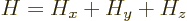where the one-di­men­sion­al Hamil­to­ni­ans are: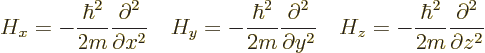To check that any prod­uct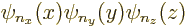of one-di­men­sion­al eigen­func­tions is an eigen­func­tion of the com­bined Hamil­ton­ian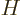, note that the par­tial Hamil­to­ni­ans only act on their own eigen­func­tion, mul­ti­ply­ing it by the cor­re­spond­ing eigen­value: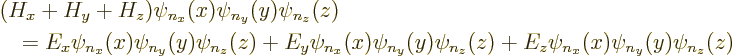or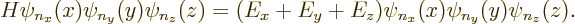There­fore, by de­f­i­n­i­tionis an eigen­func­tion of the three-di­men­sion­al Hamil­ton­ian, with an eigen­value that is the sum of the three one-di­men­sion­al ones. But there is still the ques­tion of com­plete­ness. Maybe the above eigen­func­tions are not com­plete, which would mean a need for ad­di­tional eigen­func­tions that are not prod­ucts of one-di­men­sion­al ones.

The one-di­men­sion­al eigen­func­tions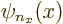are com­plete, see [41, p. 141] and ear­lier ex­er­cises in this book. So, you can write any wave func­tion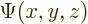at given val­ues of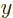and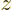as a com­bi­na­tion of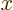-​eigen­func­tions: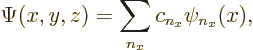but the co­ef­fi­cients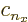will be dif­fer­ent for dif­fer­ent val­ues ofand; in other words they will be func­tions ofand: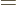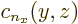. So, more pre­cisely, you have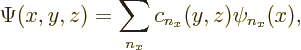But since the-​eigen­func­tions are also com­plete, at any given value of, you can write eachas a sum of-​eigen­func­tions: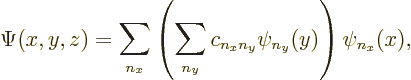where the co­ef­fi­cients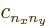will be dif­fer­ent for dif­fer­ent val­ues of,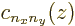. So, more pre­cisely,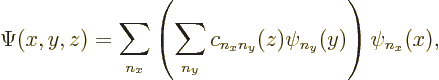But since the-​eigen­func­tions are also com­plete, you can writeas a sum of-​eigen­func­tions: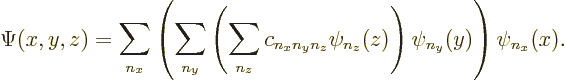Since the or­der of do­ing the sum­ma­tion does not make a dif­fer­ence,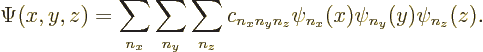So, any wave func­tioncan be writ­ten as a sum of prod­ucts of one-di­men­sion­al eigen­func­tions; these prod­ucts are com­plete.